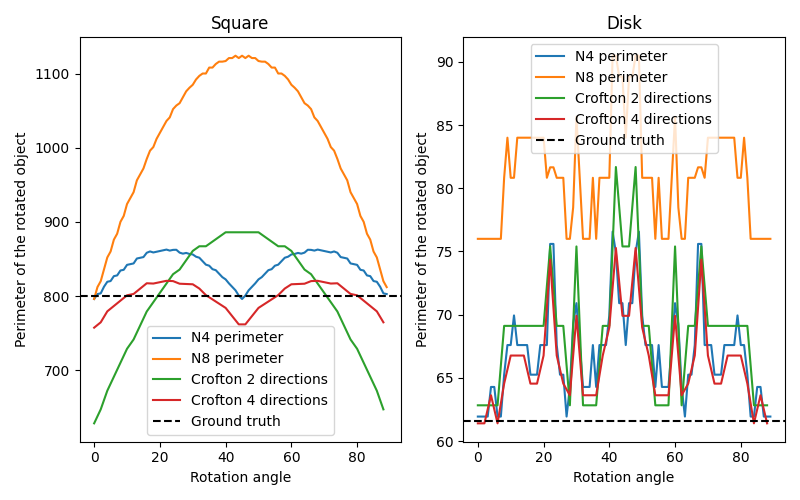# Measure perimeters with different estimators#

In this example, we show the error on measuring perimeters, comparing classic approximations and Crofton ones. For that, we estimate the perimeter of an object (either a square or a disk) and its rotated version, as we increase the rotation angle.```import matplotlib.pyplot as plt
import numpy as np

from skimage.measure import perimeter, perimeter_crofton
from skimage.transform import rotate

# scale parameter can be used to increase the grid size. The resulting curves
# should be smoothed with higher scales
scale = 10

# Construct two objects, a square and a disk
square = np.zeros((100*scale, 100*scale))
square[40*scale:60*scale, 40*scale:60*scale] = 1

[X, Y] = np.meshgrid(np.linspace(0, 100*scale), np.linspace(0, 100*scale))
R = 20 * scale
disk = (X-50*scale)**2+(Y-50*scale)**2 <= R**2

fig, axes = plt.subplots(1, 2, figsize=(8, 5))
ax = axes.flatten()

dX = X[0, 1] - X[0, 0]
true_perimeters = [80 * scale, 2 * np.pi * R / dX]

# For each type of object, estimate its perimeter as the object is rotated,
# according to different approximations
for index, obj in enumerate([square, disk]):

# `neighborhood` value can be 4 or 8 for the classic perimeter estimator
for n in [4, 8]:
p = []
angles = range(90)
for i in angles:
rotated = rotate(obj, i, order=0)
p.append(perimeter(rotated, n))
ax[index].plot(angles, p)

# `directions` value can be 2 or 4 for the Crofton estimator
for d in [2, 4]:
p = []
angles = np.arange(0, 90, 2)
for i in angles:
rotated = rotate(obj, i, order=0)
p.append(perimeter_crofton(rotated, d))
ax[index].plot(angles, p)

ax[index].axhline(true_perimeters[index], linestyle='--', color='k')
ax[index].set_xlabel('Rotation angle')
ax[index].legend(['N4 perimeter', 'N8 perimeter',
'Crofton 2 directions', 'Crofton 4 directions',
'Ground truth'],
loc='best')
ax[index].set_ylabel('Perimeter of the rotated object')

ax.set_title('Square')
ax.set_title('Disk')
plt.tight_layout()
plt.show()
```

Total running time of the script: ( 0 minutes 31.187 seconds)

Gallery generated by Sphinx-Gallery